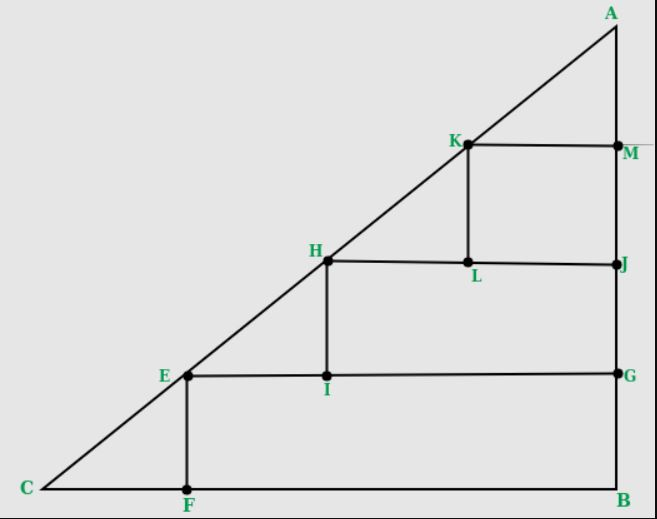# Maximum number of 2×2 squares that can be fit inside a right isosceles triangle

• Difficulty Level : Easy
• Last Updated : 29 Nov, 2021

What is the maximum number of squares of size 2×2 units that can be fit in a right-angled isosceles triangle of a given base (in units).
A side of the square must be parallel to the base of the triangle.

Examples:

Attention reader! Don’t stop learning now. Get hold of all the important DSA concepts with the DSA Self Paced Course at a student-friendly price and become industry ready.  To complete your preparation from learning a language to DS Algo and many more,  please refer Complete Interview Preparation Course.

In case you wish to attend live classes with experts, please refer DSA Live Classes for Working Professionals and Competitive Programming Live for Students.

```Input  : 8
Output : 6
Please refer below diagram for explanation.``````Input : 7
Output : 3```

Since the triangle is isosceles, the given base would also be equal to the height. Now in the diagonal part, we would always need an extra length of 2 units in both height and base of the triangle to accommodate a triangle. (The CF and AM segment of the triangle in the image. The part that does not contribute to any square). In the remaining length of base, we can construct length / 2 squares. Since each square is of 2 units, same would be the case of height, there is no need to calculate that again.
So, for each level of given length we can construct “(length-2)/2” squares. This gives us a base of “(length-2)” above it. Continuing this process to get the no of squares for all available “length-2” height, we can calculate the squares.

```while length > 2
answer += (length - 2 )/2
length = length - 2```

For more effective way, we can use the formula of sum of AP n * ( n + 1 ) / 2, where n = length – 2

## C++

 `// C++ program to count number of 2 x 2``// squares in a right isosceles triangle``#include``using` `namespace` `std;`` ` `int` `numberOfSquares(``int` `base)``{``   ``// removing the extra part we would``   ``// always need``   ``base = (base - 2);`` ` `   ``// Since each square has base of``   ``// length of 2``   ``base = ``floor``(base / 2);`` ` `   ``return` `base * (base + 1)/2;``}`` ` `// Driver code``int` `main()``{``   ``int` `base = 8;``   ``cout << numberOfSquares(base);``   ``return` `0;``}``// This code is improved by heroichitesh.`

## Java

 `// Java program to count number of 2 x 2``// squares in a right isosceles triangle`` ` `class` `Squares``{``  ``public` `static`  `int` `numberOfSquares(``int` `base)``   ``{``      ``// removing the extra part ``      ``// we would always need``      ``base = (base - ``2``);``  ` `      ``// Since each square has ``      ``// base of length of 2``      ``base = Math.floorDiv(base, ``2``);``  ` `      ``return` `base * (base + ``1``)/``2``;``   ``}``  ` `   ``// Driver code``   ``public` `static` `void` `main(String args[])``   ``{``       ` `      ``int` `base = ``8``;``      ``System.out.println(numberOfSquares(base));``   ``}``}`` ` `// This code is contributed by Anshika Goyal and improved by heroichitesh.`

## Python3

 `# Python3 program to count number``# of 2 x 2 squares in a right ``# isosceles triangle``def` `numberOfSquares(base):``     ` `    ``# removing the extra part we would``    ``# always need``    ``base ``=` `(base ``-` `2``)``     ` `    ``# Since each square has base of``    ``# length of 2``    ``base ``=` `base ``/``/` `2``     ` `    ``return` `base ``*` `(base ``+` `1``) ``/` `2``     ` `# Driver code``base ``=` `8`` ` `print``(numberOfSquares(base))`` ` `# This code is contributed by Anant Agarwal and improved by heroichitesh. `

## C#

 `// C# program to count number of 2 x 2``// squares in a right isosceles triangle``using` `System;`` ` `class` `GFG {``     ` `    ``public` `static` `int` `numberOfSquares(``int` `_base)``    ``{``         ` `        ``// removing the extra part ``        ``// we would always need``        ``_base = (_base - 2);``     ` `        ``// Since each square has ``        ``// base of length of 2``        ``_base = _base / 2;``     ` `        ``return` `_base * (_base + 1)/2;``    ``}``     ` `    ``// Driver code``    ``public` `static` `void` `Main()``    ``{``         ` `        ``int` `_base = 8;``        ``Console.WriteLine(numberOfSquares(_base));``    ``}``}`` ` `// This code is contributed by anuj_67.`

## PHP

 ``

## Javascript

 ``

Output:

`6`

This article is contributed by Harshit Agrawal. If you like GeeksforGeeks and would like to contribute, you can also write an article using write.geeksforgeeks.org or mail your article to review-team@geeksforgeeks.org. See your article appearing on the GeeksforGeeks main page and help other Geeks.